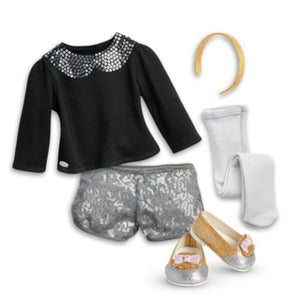# American Girl - Sparkle Spotlight Outfit For Dolls - Truly Me 2015

Vendor
American Girl
Regular price
\$64.51
Sale price
\$62.78
Quantity must be 1 or more

• S

• h

• e

• s

• p

• a

• r

• k

• l

• e

• s

• f

• r

• o

• m

• h

• e

• a

• d

• t

• o

• t

• o

• e

• a

• n

• d

• w

• i

• l

• l

• b

• e

• r

• e

• a

• d

• y

• t

• o

• s

• h

• i

• n

• e

• f

• o

• r

• a

• n

• y

• p

• a

• r

• t

• y

• o

• r

• p

• e

• r

• f

• o

• r

• m

• a

• n

• c

• e

• i

• n

• t

• h

• i

• s

• f

• u

• n

• o

• u

• t

• f

• i

• t

• !

• I

• n

• c

• l

• u

• d

• e

• s

• :

• A

• l

• o

• n

• g

• -

• s

• l

• e

• e

• v

• e

• d

• b

• l

• a

• c

• k

• t

• o

• p

• w

• i

• t

• h

• a

• P

• e

• t

• e

• r

• P

• a

• n

• f

• a

• u

• x

• -

• c

• o

• l

• l

• a

• r

• o

• f

• s

• i

• l

• v

• e

• r

• -

• c

• o

• l

• o

• r

• e

• d

• s

• e

• q

• u

• i

• n

• s

• L

• i

• n

• e

• d

• s

• h

• o

• r

• t

• s

• t

• h

• a

• t

• a

• r

• e

• c

• o

• m

• p

• l

• e

• t

• e

• l

• y

• c

• o

• v

• e

• r

• e

• d

• i

• n

• s

• h

• i

• n

• y

• s

• i

• l

• v

• e

• r

• -

• c

• o

• l

• o

• r

• e

• d

• s

• e

• q

• u

• i

• n

• s

• G

• r

• a

• y

• r

• i

• b

• -

• k

• n

• i

• t

• t

• i

• g

• h

• t

• s

• t

• h

• a

• t

• l

• o

• o

• k

• a

• d

• o

• r

• a

• b

• l

• e

• u

• n

• d

• e

• r

• h

• e

• r

• s

• h

• o

• r

• t

• s

• G

• l

• i

• t

• t

• e

• r

• y

• s

• h

• o

• e

• s

• w

• i

• t

• h

• p

• i

• n

• k

• s

• a

• t

• i

• n

• -

• r

• i

• b

• b

• o

• n

• b

• o

• w

• s

• o

• n

• t

• h

• e

• t

• o

• e

• s

• A

• h

• e

• a

• d

• b

• a

• n

• d

• t

• o

• t

• o

• p

• o

• f

• f

• h

• e

• r

• f

• a

• n

• c

• y

• l

• o

• o

• k

• P

• l

• e

• a

• s

• e

• n

• o

• t

• e

• :

• D

• o

• l

• l

• s

• o

• l

• d

• s

• e

• p

• a

• r

• a

• t

• e

• l

• y

• .

She sparkles from head to toe and will be ready to shine for any party or performance in this fun outfit! Includes: A long-sleeved black top with a Peter Pan faux-collar of silver-colored sequins Lined shorts that are completely covered in shiny silver-colored sequins Gray rib-knit tights that look adorable under her shorts Glittery shoes with pink satin-ribbon bows on the toes A headband to top off her fancy look Please note: Doll sold separately.